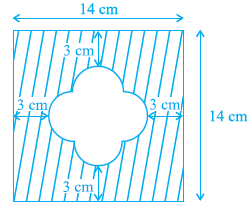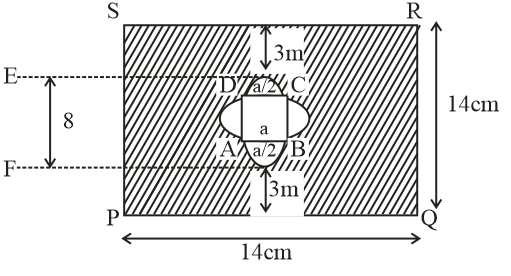#### Find the area of the shaded region given in Figure.SolutionArea of square PQRS =(side)2=(14)2

=196 cm2

Area of ABCD (let side a) =(side)2=(a)2

Area of 4 semi circle

Area of semi-circle=

Total inner area = Area of ABCD + Area of 4 semi circles

Area of inner region =

Area of shaded area = Area of PQRS – inner region area